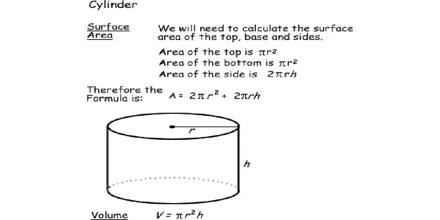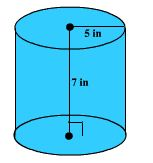Surface Area of Cylinders

Surface Area of Cylinders means the number of square units it takes to exactly cover the surface of a cylinder. To find the surface area of a cylinder adds the surface area of each end plus the surface area of the side. Each end is a circle so the surface area of each end is (π x r2), where r is the radius of the end. There are two ends so their combined surface area is (2π x r2). The surface area of the side is the circumference times the height or (2π x r x h), where r is the radius and h is the height of the side.The entire formula for the surface area of a cylinder is 2πr2 + 2 πrh

here:

• π is Pi, approximately 3.142
• r is the radius of the cylinder
• h height of the cylinder

Example: Find the total surface area of a cylinder with a base radius of 5 inches and a height of 7 inches.Solution: Total surface area = 2 π (5) (7) + 2π(52) = 120 π inches2

≈ 376.99 inches2

Units

Remember that the radius and the height must be in the same units – convert them if necessary. The resulting area will be in those square units. So, for example if the height and radius are both in centimeters, then the area will be in square centimeters.